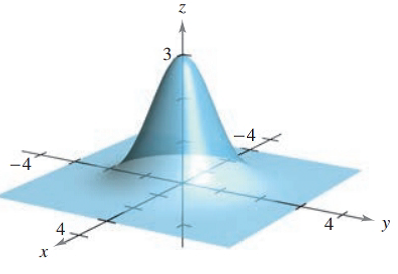Chapter 7.5, Problem 18E### Calculus: An Applied Approach (Min...

10th Edition
Ron Larson
ISBN: 9781305860919

#### Solutions

Chapter
Section### Calculus: An Applied Approach (Min...

10th Edition
Ron Larson
ISBN: 9781305860919
Textbook Problem
1 views

# Applying the Second-Partials Test In Exercises 1-18, find the relative extrema and saddle points of the function. See Examples f ( x , y ) = 3 e − ( x 2 + y 2 )To determine

To calculate: The relative extreme and saddle point of the function f(x,y)=3e(x2+y2)

Explanation

Given Information:

The equation of the function is given as f(x,y)=3e(x2+y2)

The provided figure is,

Formula used:

To check the relative extrema and saddle points

d=fxx(a,b)fyy(a,b)[fxy(a,b)]2

Following steps to be followed:

Step 1: If d>0 and fxx(a,b)>0 then the function has a relative minimum at (a, b).

Step 2: If d>0 and fxx(a,b)<0 then the function has a relative minimum at (a, b).

Step 3: If d<0 then (a,b,f(a,b)) is a saddle point.

Step 4: The best gives us information when d=0

Calculation:

Consider the given equation,

f(x,y)=3e(x2+y2)

Now, differentiate with respect to x as,

fx(x,y)={3×(2x)e(x2+y2)}fx(x,y)=6xe(x2+y2)

Now, differentiate with respect to y as,

fy(x,y)={3×(2y)e(x2+y2)}fy(x,y)=6ye(x2+y2)

So, fx(x,y) and fy(x,y) are defined for all points in the xy-plane

### Still sussing out bartleby?

Check out a sample textbook solution.

See a sample solution

#### The Solution to Your Study Problems

Bartleby provides explanations to thousands of textbook problems written by our experts, many with advanced degrees!

Get Started

#### Calculate y'. 20. y = exsec x

Single Variable Calculus: Early Transcendentals, Volume I

#### In Exercises 4143, find the distance between the two points. 43. (12,3) and (12,23)

Applied Calculus for the Managerial, Life, and Social Sciences: A Brief Approach

#### CHECK POINT Decide which of the following are undefined. 1.

Mathematical Applications for the Management, Life, and Social Sciences

#### = _____. 0 does not exist

Study Guide for Stewart's Single Variable Calculus: Early Transcendentals, 8th

#### Given the Taylor Series , a Taylor series for ex/2 is:

Study Guide for Stewart's Multivariable Calculus, 8th